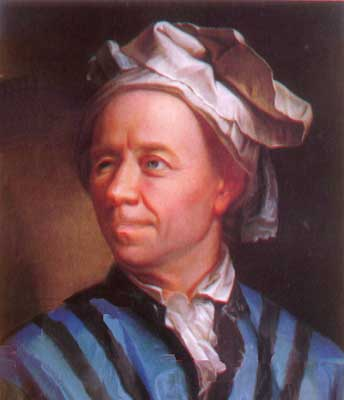# First Order Differential Equation SolverLeonhard Euler
(Image source)

This program will allow you to obtain the numerical solution to the first order initial value problem:

 dy/dt = f(t,y)          on [t0, t1] y(t0) = y0

using one of three different methods; Euler's method, Heun's method (also known as the improved Euler method), and a fourth-order Runge-Kutta method. To solve a problem, choose a method, fill in the fields below, choose the output format, and then click on the "Submit" button.

A wide range of functions, e.g. exp(t) and sinh(t), are supported and whitespace is allowed. Mathematical expressions are entered just as they would be in most programming languages:

• use * for multiply,
• use / for divide, and
• use ^ or ** to raise a quantity to a power.

For example, the function (1+y2)etsin(2t) could be entered in either of the following two ways:

• (1+y^2)*exp(-t)*sin(2*t),
• (1+y**2)*exp(-t)*sin(2*t).

 Method Euler (Tangent Line) Heun (Improved Euler) 4th Order Runge-Kutta dy/dt = f(t,y) = t0 = y0 = t1 = Step size h = Output format Graph only Data points only Graph and Data points